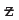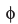Test: Theoretical Distributions- 3

# Test: Theoretical Distributions- 3

Test Description

## 40 Questions MCQ Test Business Mathematics and Logical Reasoning & Statistics | Test: Theoretical Distributions- 3

Test: Theoretical Distributions- 3 for CA Foundation 2022 is part of Business Mathematics and Logical Reasoning & Statistics preparation. The Test: Theoretical Distributions- 3 questions and answers have been prepared according to the CA Foundation exam syllabus.The Test: Theoretical Distributions- 3 MCQs are made for CA Foundation 2022 Exam. Find important definitions, questions, notes, meanings, examples, exercises, MCQs and online tests for Test: Theoretical Distributions- 3 below.
Solutions of Test: Theoretical Distributions- 3 questions in English are available as part of our Business Mathematics and Logical Reasoning & Statistics for CA Foundation & Test: Theoretical Distributions- 3 solutions in Hindi for Business Mathematics and Logical Reasoning & Statistics course. Download more important topics, notes, lectures and mock test series for CA Foundation Exam by signing up for free. Attempt Test: Theoretical Distributions- 3 | 40 questions in 40 minutes | Mock test for CA Foundation preparation | Free important questions MCQ to study Business Mathematics and Logical Reasoning & Statistics for CA Foundation Exam | Download free PDF with solutions
 1 Crore+ students have signed up on EduRev. Have you?
Test: Theoretical Distributions- 3 - Question 1

### Q. The number of accidents in a year attributed to taxi drivers in a locality follows Poisson distribution with an average 2. Out of 500 taxi drivers of that area, what is the number of drivers with at least 3 accidents in a year?

Test: Theoretical Distributions- 3 - Question 2

### In a sample of 800 students, the mean weight and standard deviation of weight are found to be 50 Kg and 20 Kg respectively. On the assumption of normality, what is the number of students weighing between 46 Kg and 62 Kg? Given area of the standard normal curve between= 0 to= 0.20 = 0.0793 and area between= 0 to= 0.60 = 0.2257.

Test: Theoretical Distributions- 3 - Question 3

### The salary of workers of a factory is known to follow normal distribution with an average salary of Rs. 10,000 and standard deviation of salary as Rs. 2,000. If 50 workers receive salary more than Rs. 14,000, then the total no. of workers in the factory is

Test: Theoretical Distributions- 3 - Question 4

For a normal distribution with mean as 500 and SD as 120, what is the value of k so that the interval [500, k] covers 40.32 per cent area of the normal curve? Given(1.30) = 0.9032.

Test: Theoretical Distributions- 3 - Question 5

The average weekly food expenditure of a group of families has a normal distribution with mean Rs. 1,800 and standard deviation Rs. 300. What is the probability that out of 5 families belonging to this group, at least one family has weekly food expenditure in excess of Rs. 1,800? Given(1) = 0.84.

Test: Theoretical Distributions- 3 - Question 6

If the weekly wages of 5000 workers in a factory follows normal distribution with mean and SD as Rs. 700 and Rs. 50 respectively, what is the expected number of workers with wages between Rs. 660 and Rs. 720?

Test: Theoretical Distributions- 3 - Question 7

50 per cent of a certain product have weight 60 Kg or more whereas 10 per cent have weight 55 Kg or less. On the assumption of normality, what is the variance of weight?Given(1.28) = 0.90.

Test: Theoretical Distributions- 3 - Question 8

When a coin is tossed 10 times then

Test: Theoretical Distributions- 3 - Question 9

In Binomial Distribution ‘n‘ means

Test: Theoretical Distributions- 3 - Question 10

Binomial Distribution is a

Test: Theoretical Distributions- 3 - Question 11

When there are a fixed number of repeated trial of any experiments under identical conditions for which only one of two mutually exclusive outcomes, success or failure can result in each trial then

Test: Theoretical Distributions- 3 - Question 12

In Binomial Distribution ‘p’ denotes Probability of

Test: Theoretical Distributions- 3 - Question 13

When ‘p’ = 0.5, the binomial distribution is

Test: Theoretical Distributions- 3 - Question 14

When ‘p’ is larger than 0. 5, the binomial distribution is

Test: Theoretical Distributions- 3 - Question 15

Mean of Binomial distribution is

Test: Theoretical Distributions- 3 - Question 16

Variance of Binomial distribution is

Test: Theoretical Distributions- 3 - Question 17

When p = 0.1 the binomial distribution is skewed to the

Test: Theoretical Distributions- 3 - Question 18

If in Binomial distribution np = 9 and npq = 2. 25 then q is equal to

Test: Theoretical Distributions- 3 - Question 19

In Binomial Distribution

Test: Theoretical Distributions- 3 - Question 20

Standard deviation of binomial distribution is

Test: Theoretical Distributions- 3 - Question 21

_________ distribution is a limiting case of Binomial distribution

Test: Theoretical Distributions- 3 - Question 22

When the no. of trials is large then

Test: Theoretical Distributions- 3 - Question 23

In Poisson Distribution, probability of success is very close to

Test: Theoretical Distributions- 3 - Question 24

In Poisson Distribution np is

Test: Theoretical Distributions- 3 - Question 25

In ________________ distribution, mean = variance

Test: Theoretical Distributions- 3 - Question 26

In Poisson distribution mean is equal to

Test: Theoretical Distributions- 3 - Question 27

In Poisson distribution standard deviation is equal to

Test: Theoretical Distributions- 3 - Question 28

For continuous events _________________ distribution is used.

Test: Theoretical Distributions- 3 - Question 29

For continuous events _________________ distribution is used.

Detailed Solution for Test: Theoretical Distributions- 3 - Question 29

The probability density function (pdf) of the normal distribution, also called Gaussian or "bell curve", the most important continuous random distribution.

Test: Theoretical Distributions- 3 - Question 30

Probability density function is always

Test: Theoretical Distributions- 3 - Question 31

In continuous cases probability of the entire space is

Test: Theoretical Distributions- 3 - Question 32

In discrete case the probability of the entire space is

Test: Theoretical Distributions- 3 - Question 33

Binomial distribution is symmetrical if

Test: Theoretical Distributions- 3 - Question 34

The Poisson distribution tends to be symmetrical if the mean value is

Test: Theoretical Distributions- 3 - Question 35

The curve of ____________ distribution has single peak

Test: Theoretical Distributions- 3 - Question 36

The curve of _________ distribution is unimodal and bell shaped with the highest point over the mean

Test: Theoretical Distributions- 3 - Question 37

Because of the symmetry of Normal distribution the median and the mode have the ______ value as that of the mean

Test: Theoretical Distributions- 3 - Question 38

For a Normal distribution, the total area under the normal curve is

Test: Theoretical Distributions- 3 - Question 39

In Normal distribution the probability has the maximum value at the

Test: Theoretical Distributions- 3 - Question 40

In Normal distribution the probability decreases gradually on either side of the mean but never touches the axis.

## Business Mathematics and Logical Reasoning & Statistics

81 videos|101 docs|87 tests
 Use Code STAYHOME200 and get INR 200 additional OFF Use Coupon Code
Information about Test: Theoretical Distributions- 3 Page
In this test you can find the Exam questions for Test: Theoretical Distributions- 3 solved & explained in the simplest way possible. Besides giving Questions and answers for Test: Theoretical Distributions- 3, EduRev gives you an ample number of Online tests for practice

## Business Mathematics and Logical Reasoning & Statistics

81 videos|101 docs|87 tests